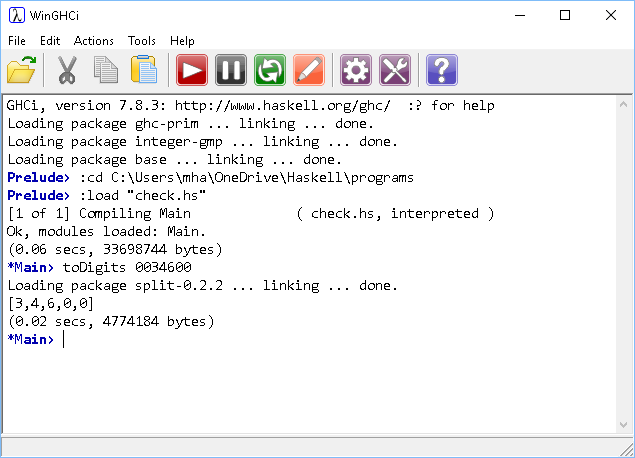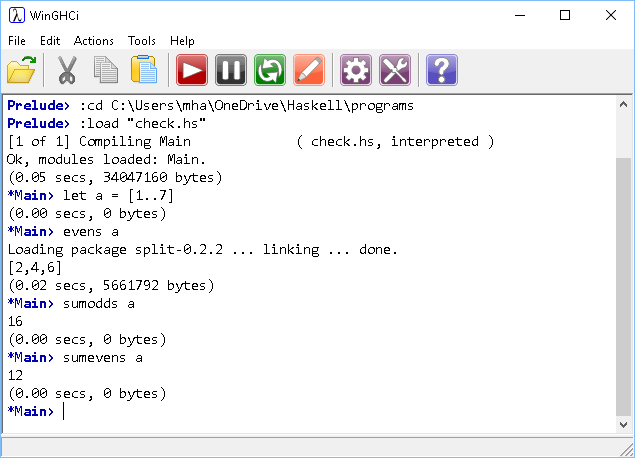# Introduction To Haskell Check Digits

## Introduction

The bar codes on products are machine-readable representations of the human-readable digits shown below the bars. Two typical standards for bar code numbers are European Article Number 8 (EAN8) and EAN13. Following these standards, the last digit of the bar code is calculated from the previous ones. Since computers tend to be attached to the devices that read bar codes, this provides a simple calculation that can eliminate a large proportion of reading errors.

The system used on the EAN8 bar codes is as follows,

The last digit is chosen so that,

3 x (1st + 3rd + 5th + 7th number) + (2nd + 4th +6th +8th number)

divides exactly by 10.

Take the code 0034 600.

```1st + 3rd + 5th + 7th number = 0 + 3 + 6 + 0 = 9. Multiplying by 3 gives 3 x 9 = 27. 27 + 2nd + 4th + 6th number = 27 + 0 + 4 + 0 = 31. In order to make the total divisible by 10, we need to add another 9. The check digit is therefore 9.```

Our task on this page is to write a program that will calculate the check digit for a given 7 digit code.

## Programming - Generating The Check Digit

We can divide this task into stages, each one needing a solution before it makes sense to work on the next step. My solution to this problem is made up of the following steps,

1. Convert the 7 digit code to a list of its digits.
2. Make and sum a list of the odd-positioned digits.
3. Make and sum a list of the even-positioned digits.
4. Work out the check digit.

### Digit List

I reuse this function quite a lot. It uses division by 10 to separate the digits into a list.

```toDigits n
| n < 1 = []
| otherwise = toDigits (div n 10) ++ [mod n 10]
```

The function is recursive. It divides the number by 10, placing the remainder at the end of the list and supplying the quotient as the argument in a recursive call. It stops when the number is less than 1.

There is a problem though. Suppose we want to try out the number used in the example at the top of the page. This is what we get,If there is an odd number of 0 digits at the start of the pattern, they are discarded when we store the information as an integer. We have options here but the simplest catch-all is to prepend 0 to the start of the list until it is of length 7.

This version includes a fix for that and a new function composing the process for us nicely,

```toDigits n
| n < 1 = []
| otherwise = toDigits (div n 10) ++ [mod n 10]

makeSeven xs = replicate (7 - length xs) 0 ++ xs

toSevenDigits = makeSeven . toDigits
```

We replicate as many zero digits as we need to get the length up to 7. This ensures that insignificant 0s are not lost in the integer representation.

### Odd-Positioned Digits

There are quite a few ways to do this. There are some functions in the Data library that could help here. It's worth getting our own version though.

```odds [] = []
odds [x] = [x]
odds (x:y:xs) = x: odds xs
```

If the argument is an empty list, an empty list is returned. If there is a single item in the list, it is returned as a list. If not, a list is returned. The head of that list is the head of the argument list. The tail of that list is formed with a recursive call on the tail of the tail of the argument list.### Even-Positioned Digits

This is pretty simple if we just drop the first item of the list and use our previous function. We might as well define some nicely named functions for the summation too.

```evens l = odds (drop 1 l)

sumodds = sum . odds
sumevens = sum . evens
```

Testing,### Check Digit

Now we get to use our functions to make our check digit generator,

```generateEAN8 n = if rem ==0 then 0 else (10 - rem)
where
xs = toSevenDigits n
odds = sumodds xs
evens = sumevens xs
tot = 3 * odds + evens
rem = mod tot 10
```
Haskell allows an if..then..else structure. In this function, the if is used to express the final decision of which digit to allocate. The variable rem is the remainder when we divide that total by 10. The where is used to define the steps of the calculation.### Whole Program

There are probably more efficient ways to do this. It is clearly possible to combine many of the functions, indeed all of them. There is always a trade-off to be had between more efficient execution, shorter code and having a program that is easy to follow.

```toDigits n
| n < 1 = []
| otherwise = toDigits (div n 10) ++ [mod n 10]

makeSeven xs = replicate (7 - length xs) 0 ++ xs

toSevenDigits = makeSeven . toDigits

odds [] = []
odds [x] = [x]
odds (x:y:xs) = x: odds xs

evens l = odds (drop 1 l)

sumodds = sum . odds
sumevens = sum . evens

generateEAN8 n = if rem ==0 then 0 else (10 - rem)
where
xs = toSevenDigits n
odds = sumodds xs
evens = sumevens xs
tot = 3 * odds + evens
rem = mod tot 10
```

## Challenges

1. The first challenge is to extend the code so that you can validate a check digit. You need to make sure to left pad the argument to ensure that you have 8 digits. Your validation function can be very similar to the generator. Instead of returning the check digit, it returns true or false.
2. For EAN13 bar codes, which use the same system as ISB13, you use a very similar algorithm. This time, the even-positioned items are multiplied by 3 and added to the sum of the odds. It's an easy modification of the example code to get a generator for EAN13.
3. ISBN10 is no more, but you still see the bar codes on old books. Research the system for that and implement your own program.